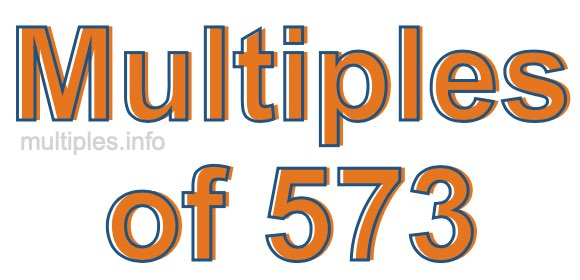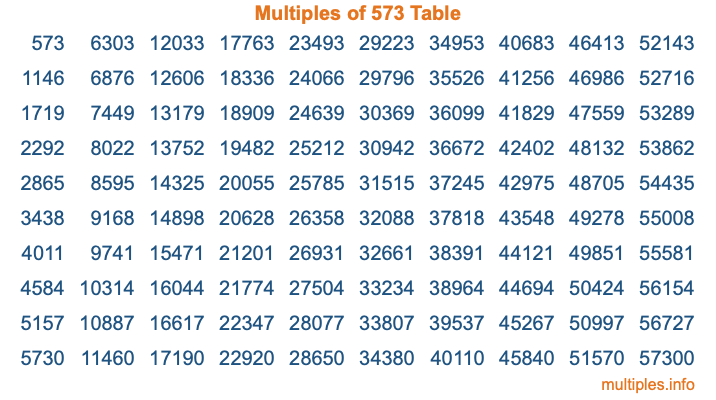Multiples of 573Welcome to the Multiples of 573 page. Here we will first teach you everything you will ever need to know about the multiples of 573, and then give you a study guide summary of everything we taught you to make sure you remember it all. Use this page to look up facts and learn information about the multiples of 573. This page will make you a multiples of five hundred seventy-three expert!

Definition of Multiples of 573
Multiples of 573 are all the numbers that when divided by 573 equal an integer. Each of the multiples of 573 are called a multiple. A multiple of 573 is created by multiplying 573 by an integer.

Therefore, to create a list of multiples of 573, you start with 1 multiplied by 573, then 2 multiplied by 573, then 3 multiplied by 573, and so on for as long as you want. Thus, the list of the first five multiples of 573 is 573, 1146, 1719, 2292, and 2865. To see a larger list of multiples of 573, see the printable image of Multiples of 573 further down on this page. We also have a category where you can choose any nth multiple of 573.

Multiples of 573 Checker
The Multiples of 573 Checker below checks to see if any number of your choice is a multiple of 573. In other words, it checks to see if there is any number (integer) that when multiplied by 573 will equal your number. To do that, we divide your number by 573. If the the quotient is an integer, then your number is a multiple of 573.

Is  a multiple of 573?

Least Common Multiple of 573 and ...
A Least Common Multiple (LCM) is the lowest multiple that two or more numbers have in common. This is also called the smallest common multiple or lowest common multiple and is useful to know when you are adding our subtracting fractions. Enter one or more numbers below (573 is already entered) to find the LCM.

Check out our LCM Calculator if you need more details about the Least Common Multiple or if you need the LCM for different numbers for adding and subtraction fractions.

nth Multiple of 573
As we stated above, 573 is the first multiple of 573, 1146 is the second multiple of 573, 1719 is the third multiple of 573, and so on. Enter a number below to find the nth multiple of 573.

th multiple of 573

Multiples of 573 vs Factors of 573
573 is a multiple of 573 and a factor of 573, but that is where the similarities end. All postive multiples of 573 are 573 or greater than 573. All positive factors of 573 are 573 or less than 573.

Below is the beginning list of multiples of 573 and the factors of 573 so you can compare:

Multiples of 573: 573, 1146, 1719, 2292, 2865, etc.

Factors of 573: 1, 3, 191, 573

As you can see, the multiples of 573 are all the numbers that you can divide by 573 to get a whole number. The factors of 573, on the other hand, are all the whole numbers that you can multiply by another whole number to get 573.

It's also interesting to note that if a number (x) is a factor of 573, then 573 will also be a multiple of that number (x).

Multiples of 573 vs Divisors of 573
The divisors of 573 are all the integers that 573 can be divided by evenly. Below is a list of the divisors of 573.

Divisors of 573: 1, 3, 191, 573

The interesting thing to note here is that if you take any multiple of 573 and divide it by a divisor of 573, you will see that the quotient is an integer.

Multiples of 573 Table
Below is an image of the first 100 multiples of 573 in a table. The table is in chronological order, column by column. The first column has the first ten multiples of 573, the second column has the next ten multiples of 573, and so on.The Multiples of 573 Table is also referred to as the 573 Times Table or Times Table of 573. You are welcome to print out our table for your studies.

Negative Multiples of 573
Although not often discussed or needed in math, it is worth mentioning that you can make a list of negative multiples of 573 by multiplying 573 by -1, then by -2, then by -3, and so on, to get the following list of negative multiples of 573:

-573, -1146, -1719, -2292, -2865, etc.

Multiples of 573 Summary
Below is a summary of important Multiples of 573 facts that we have discussed on this page. To retain the knowledge on this page, we recommend that you read through the summary and explain to yourself or a study partner why they hold true.

There are an infinite number of multiples of 573.

A multiple of 573 divided by 573 will equal a whole number.

573 divided by a factor of 573 equals a divisor of 573.

The nth multiple of 573 is n times 573.

The largest factor of 573 is equal to the first positive multiple of 573.

573 is a multiple of every factor of 573.

573 is a multiple of 573.

A multiple of 573 divided by a divisor of 573 equals an integer.

573 divided by a divisor of 573 equals a factor of 573.

Any integer times 573 will equal a multiple of 573.

Multiples of a Number
Here you can get the multiples of another number, all with the same attention to detail as we did for multiples of 573 on this page.

Multiples of
Multiples of 574
Did you find our page about multiples of five hundred seventy-three educational? Do you want more knowledge? Check out the multiples of the next number on our list!